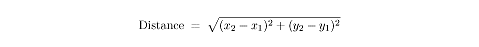# SAT数学分析：Lines and Distance

来源：新东方在线    要点：SAT数学分析Lines and distance are fundamental to coordinate geometry, not to mention to the Math IC test. Even the most complicated coordinate geometry question uses the concepts covered in the next few sections.

Distance Measuring distance in the coordinate plane is made possible thanks to the Pythagorean theorem. If you are given two points, (x1,y1), and (x2,y2), their distance from each other is given by the following formula:The diagram below shows how the Pythagorean theorem plays a role in the formula. The distance between two points can be represented by the hypotenuse of a right triangle whose legs are lengths (x2 – x1) and (y2 – y1).To calculate the distance from (4, –3) to (–3, 8), plug the coordinates into the formula:The distance between the points is , which equals approximately 13.04. You can double-check this answer by plugging it back into the Pythgorean theorem.Finding Midpoints The midpoint between two points in the coordinate plane can be calculated using a formula. If the endpoints of a line segment are (x1, y1) and (x2, y2), then the midpoint of the line segment is:In other words, the x- and y-coordinates of the midpoint are the averages of the x- and y-coordinates of the endpoints.Here’s a practice question:What is the midpoint of the line segment whose endpoints are (6, 0) and (3, 7)?　　To solve, all you need to do is plug the points given into the midpoint formula . x1 = 6, y1 = 0, x2 = 3, and y2 = 7: